# 布隆过滤器简介

1. 占用的存储空间较小。不需要像 HashSet 一样存储 Key 的原始数据。

1. 存在误判率，过滤器认为不存在的数据一定不存在，但是认为存在的数据不一定真的存在。这个和布隆过滤器的实现方式有关。
2. 不支持数据的删除，下文会讲为什么不支持删除。

## Hash 冲突的解决方案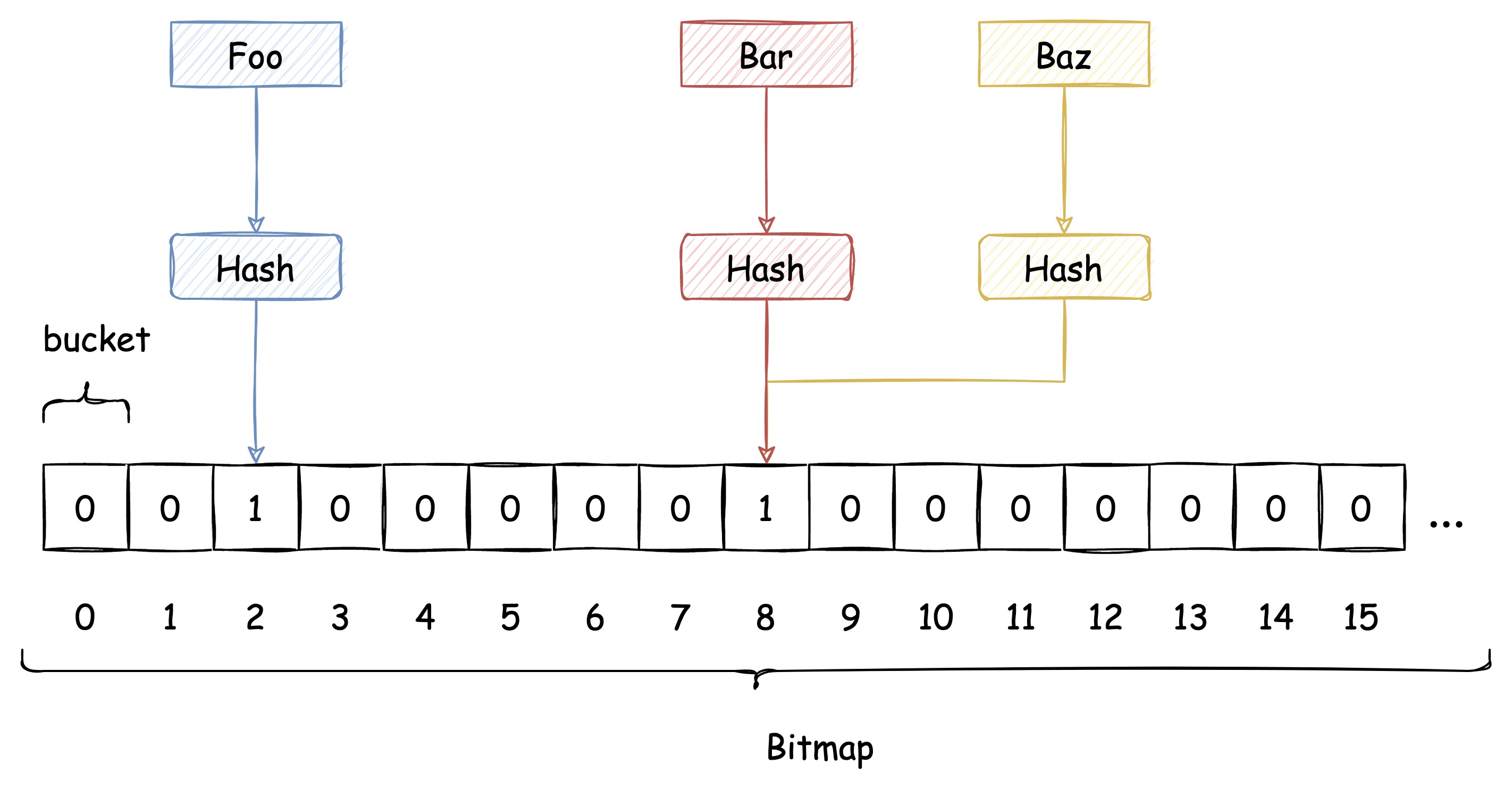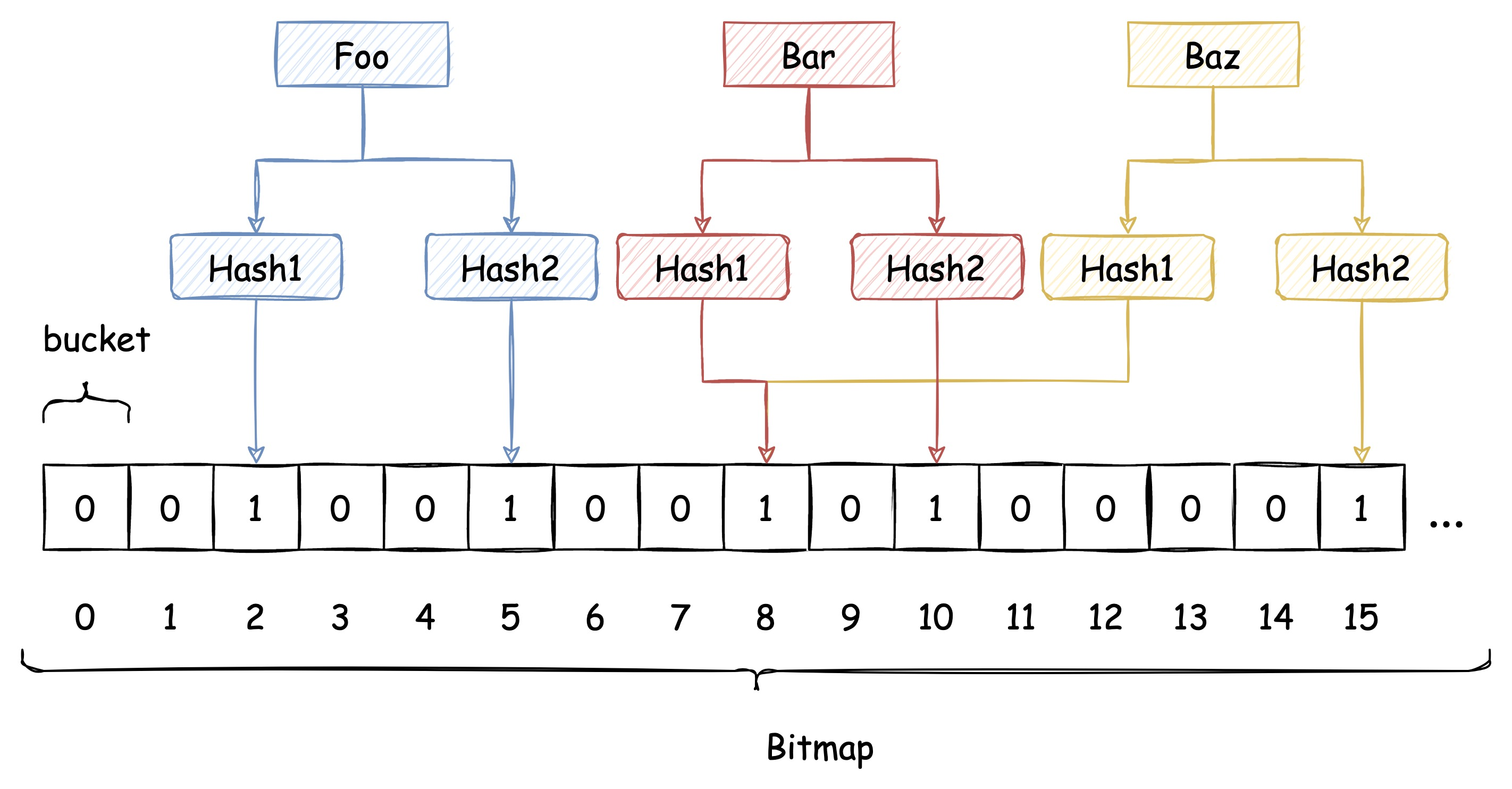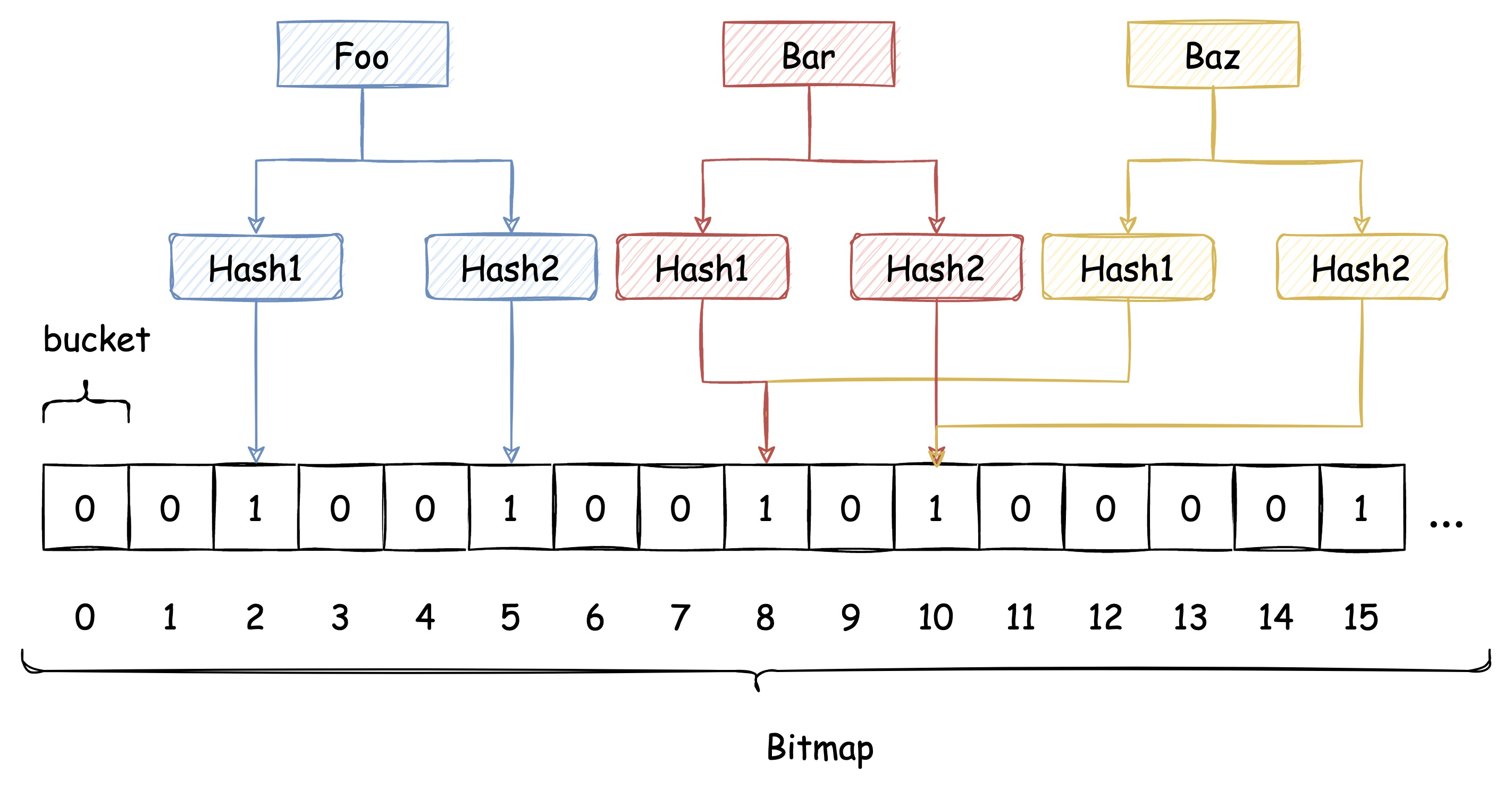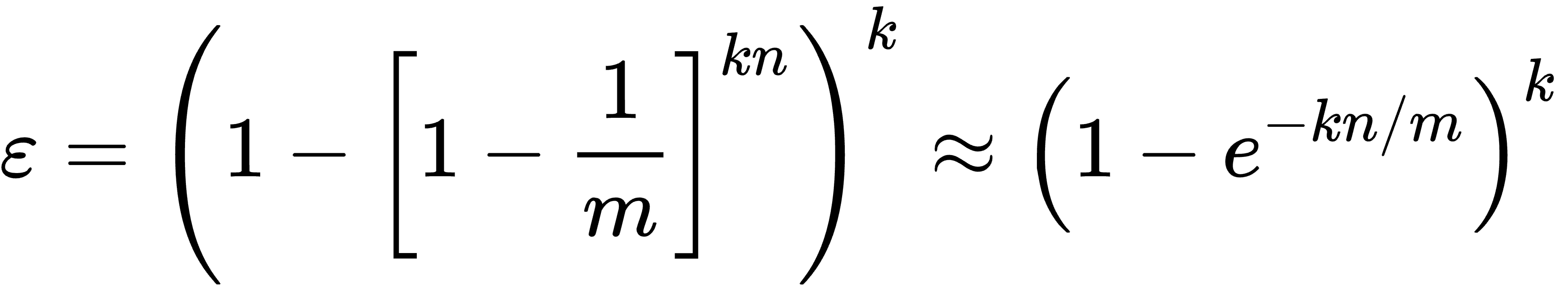# 用 C# 实现 Bitmap

## 位运算

& 两个位都为1时，结果才为1
| 两个位都为0时，结果才为0
^ 异或 两个位相同为0，相异为1
~ 取反 0变1，1变0
<< 左移 各二进位全部左移若干位，低位补0
>> 右移 各二进位全部右移若干位，高位补0

// 0b 代表值用二进制表示数字
short a =                     0b0111111111111001;
byte  b =                             0b01111111;
short c = (short)(a & b);  // 0b0000000001111001
short d = (short)(a | b);  // 0b0111111111111111
short e = (short)(a ^ b);  // 0b0111111110000110
byte  f = (byte)~b;        //         0b10000000
short g = (short)(b << 1); // 0b0000000011111110
short h = (short)(b >> 1); // 0b0000000000111111


## 利用位运算创建 Bitmap

1. 指定位设置成 1
按前面说的位运算的规则，是不能够单独修改 bit 序列中某一位的。位运算需要从右到左一对对计算。
使用 | 可以实现这个功能。假设我们要改变从右开始下标为 3（初始位置0） 的 bit 的值，则需要准备一个该位置为 1，其他位置都是 0 的 bit 序列，与要改变的 bit 序列进行 | 运算。
// 为了将 a 的右边数起第 3 位改成 1，需要准备一个 b
byte a =            0b010100010;
byte b = 1 << 3; // 0b000001000
a |= b;          // 0b010101010

1. 指定位设置成 0
和设置成 1 正好相反，需要准备一个指定位置为 0，其他位置都是 1 的 bit 序列，与要改变的 bit 序列进行 & 运算。
byte a =            0b010101010;
byte b = 1 << 3; // 0b000001000
b = ~b;          // 0b111110111
a &= b;          // 0b010100010

1. 查看指定位的值
利用 & 运算符，只要计算结果不为 0，就代表指定位置的值为 1。
byte a =            0b010101010;
byte b = 1 << 3; // 0b000001000;
a &= b;          // 0b000001000;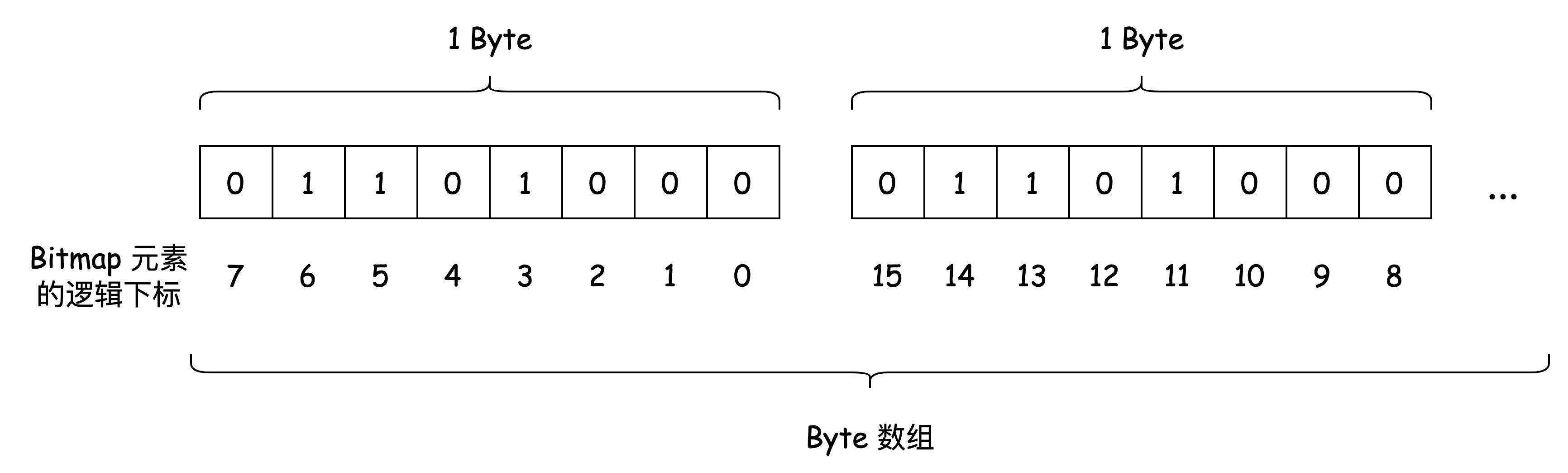class Bitmap
{

public Bitmap(long capacity)
{
_capacity = capacity;
_bytes = new byte[_capacity / 8 + 1];
}

public long Capacity => _capacity;

public void Set(long index)
{
if (index >= _capacity)
{
throw new IndexOutOfRangeException();
}

// 计算出数据存在第几个 byte 上
long byteIndex = index / 8;
// 计算出数据存在第几个 bit 上
int bitIndex = (int)(index % 8);
_bytes[byteIndex] |= (byte)(1 << bitIndex);
}

public void Remove(long index)
{
if (index >= _capacity)
{
throw new IndexOutOfRangeException();
}

long byteIndex = index / 8;
int bitIndex = (int)(index % 8);
_bytes[byteIndex] &= (byte)~(1 << bitIndex);
}

public bool Get(long index)
{
if (index >= _capacity)
{
throw new IndexOutOfRangeException();
}

long byteIndex = index / 8;
int bitIndex = (int)(index % 8);

return (_bytes[byteIndex] & (byte)(1 << bitIndex)) != 0;
}
}


# 用 C# 实现 布隆过滤器

## MurmurHash3 的使用

https://github.com/darrenkopp/murmurhash-net

byte[] data = Guid.NewGuid().ToByteArray();
// returns a 128-bit algorithm using "unsafe" code with default seed
HashAlgorithm murmur128 = MurmurHash.Create128(managed: false);
byte[] hash = murmur128.ComputeHash(data);


## 将任意类型的 key 转换为 byte 数组

### Funnel 与 Sink 的定义

1. Funnel：将各类数据转换成 byte 数组，包括 int、bool、string 等built-in 类型及自定义的复杂类型。

2. Sink：Funnel 的核心组件，作为数据的缓冲区。Funnel 在将自定义的复杂类型实例转换成 byte 数组时，就需要将数据拆解分批写入 sink。

Funnel 可以定义成如下的委托，接受原始值，并将其写入 sink 中。

delegate void Funnel<in T>(T from, ISink sink);


Sink 将不同类型的数据转换成 byte 数组并汇总到一起。

interface ISink
{
ISink PutByte(byte b);

ISink PutBytes(byte[] bytes);

ISink PutBool(bool b);

ISink PutShort(short s);

ISink PutInt(int i);

ISink PutString(string s, Encoding encoding);

ISink PutObject<T>(T obj, Funnel<T> funnel);

/// ... 其他 built-in 类型，读者可自行补充
}


public class Funnels
{
public static Funnel<string> StringFunnel = (from, sink) =>
sink.PutString(from, Encoding.UTF8);

public static Funnel<int> IntFunnel = (from, sink) =>
sink.PutInt(from);
}


Funnel<Foo> funnelFoo = (foo, sink) =>
{
sink.PutString(foo.A, Encoding.UTF8);
sink.PutInt(foo.B);

Funnel<Bar> funnelBar = (bar, barSink) => barSink.PutBool(bar.C);
sink.PutObject(foo.Bar, funnelBar);
};

class Foo
{
public string A { get; set; }

public int B { get; set; }

public Bar Bar { get; set; }
}

class Bar
{
public bool C { get; set; }
}


### Sink 的实现

Sink 的核心是 byte 数组缓冲区的实现，利用 ArrayPool 我们可以很方便的实现一个 ByteBuffer。

class ByteBuffer : IDisposable
{
private int _offset;
private bool _disposed;

public ByteBuffer(int capacity)
{
_capacity = capacity;
_buffer = ArrayPool<byte>.Shared.Rent(capacity);
}

public void Put(byte b)
{
CheckInsertable();
_buffer[_offset] = b;
_offset++;
}

public void Put(byte[] bytes)
{
CheckInsertable();
bytes.CopyTo(_buffer.AsSpan(_offset, bytes.Length));
_offset += bytes.Length;
}

public void PutInt(int i)
{
CheckInsertable();
BinaryPrimitives.WriteInt32BigEndian(GetRemainingAsSpan(), i);
_offset += sizeof(int);
}

public void PutShort(short s)
{
CheckInsertable();
BinaryPrimitives.WriteInt32BigEndian(GetRemainingAsSpan(), s);
_offset += sizeof(short);
}

// ... 其他的 primitive type 的实现

public Span<byte> GetBuffer() =>
_buffer.AsSpan(.._offset);

public bool HasRemaining() => _offset < _capacity;

public void Dispose()
{
_disposed = true;
ArrayPool<byte>.Shared.Return(_buffer);
}

private void CheckInsertable()
{
if (_disposed)
{
throw new ObjectDisposedException(typeof(ByteBuffer).FullName);
}

if (_offset >= _capacity)
{
throw new OverflowException("Byte buffer overflow");
}
}

private Span<byte> GetRemainingAsSpan() => _buffer.AsSpan(_offset..);
}


Sink 则是对 ByteBuffer 的进一步封装，来适配当前使用场景。

class Sink : ISink, IDisposable
{

/// <summary>
/// 创建一个新的 <see cref="Sink"/> 实例
/// </summary>
/// <param name="expectedInputSize">预计输入的单个元素的最大大小</param>
public Sink(int expectedInputSize)
{
_byteBuffer = new ByteBuffer(expectedInputSize);
}

public ISink PutByte(byte b)
{
_byteBuffer.Put(b);
return this;
}

public ISink PutBytes(byte[] bytes)
{
_byteBuffer.Put(bytes);
return this;
}

public ISink PutBool(bool b)
{
_byteBuffer.Put((byte)(b ? 1 : 0));
return this;
}

public ISink PutShort(short s)
{
_byteBuffer.PutShort(s);
return this;
}

public ISink PutInt(int i)
{
_byteBuffer.PutInt(i);
return this;
}

public ISink PutString(string s, Encoding encoding)
{
_byteBuffer.Put(encoding.GetBytes(s));
return this;
}

public ISink PutObject<T>(T obj, Funnel<T> funnel)
{
funnel(obj, this);
return this;
}

public byte[] GetBytes() => _byteBuffer.GetBuffer().ToArray();

public void Dispose()
{
_byteBuffer.Dispose();
}
}


## k 个 Hash 函数与 布隆过滤器 实现

hash(k) = hash1 + (k-1) * hash2

public class BloomFilter<T>
{

/// <summary>
/// 创建一个新的 <see cref="BloomFilter"/> 实例
/// </summary>
/// <param name="funnel">与插入元素类型相关的<see cref="Funnel"/>的实现</param>
/// <param name="buckets">BloomFilter 内部 Bitmap 的 bucket 数量，越大，误判率越低</param>
/// <param name="hashFunctions">hash 函数的数量，越多，误判率越低</param>
/// <param name="expectedInputSize">预计插入的单个元素的最大大小</param>
public BloomFilter(Funnel<T> funnel, int buckets, int hashFunctions = 2, int expectedInputSize = 128)
{
_hashFunctions = hashFunctions;
_funnel = funnel;
_expectedInputSize = expectedInputSize;

_bitmap = new Bitmap(buckets);
_murmur128 = MurmurHash.Create128(managed: false);
}

{
long bitSize = _bitmap.Capacity;

var (hash1, hash2) = Hash(item);

long combinedHash = hash1;
for (int i = 0; i < _hashFunctions; i++)
{
_bitmap.Set((combinedHash & long.MaxValue) % bitSize);
combinedHash += hash2;
}
}

public bool MightContains(T item)
{
long bitSize = _bitmap.Capacity;

var (hash1, hash2) = Hash(item);

long combinedHash = hash1;
for (int i = 0; i < _hashFunctions; i++)
{
if (!_bitmap.Get((combinedHash & long.MaxValue) % bitSize))
{
return false;
}

combinedHash += hash2;
}

return true;
}

[MethodImpl(MethodImplOptions.AggressiveInlining)]
private (long Hash1, long Hash2) Hash(T item)
{
byte[] inputBytes;
using (var sink = new Sink(_expectedInputSize))
{
sink.PutObject(item, _funnel);
inputBytes = sink.GetBytes();
}

var hashSpan = _murmur128.ComputeHash(inputBytes).AsSpan();

return (lowerEight, upperEight);
}
}


# 扩展

## 带计数器的布隆过滤器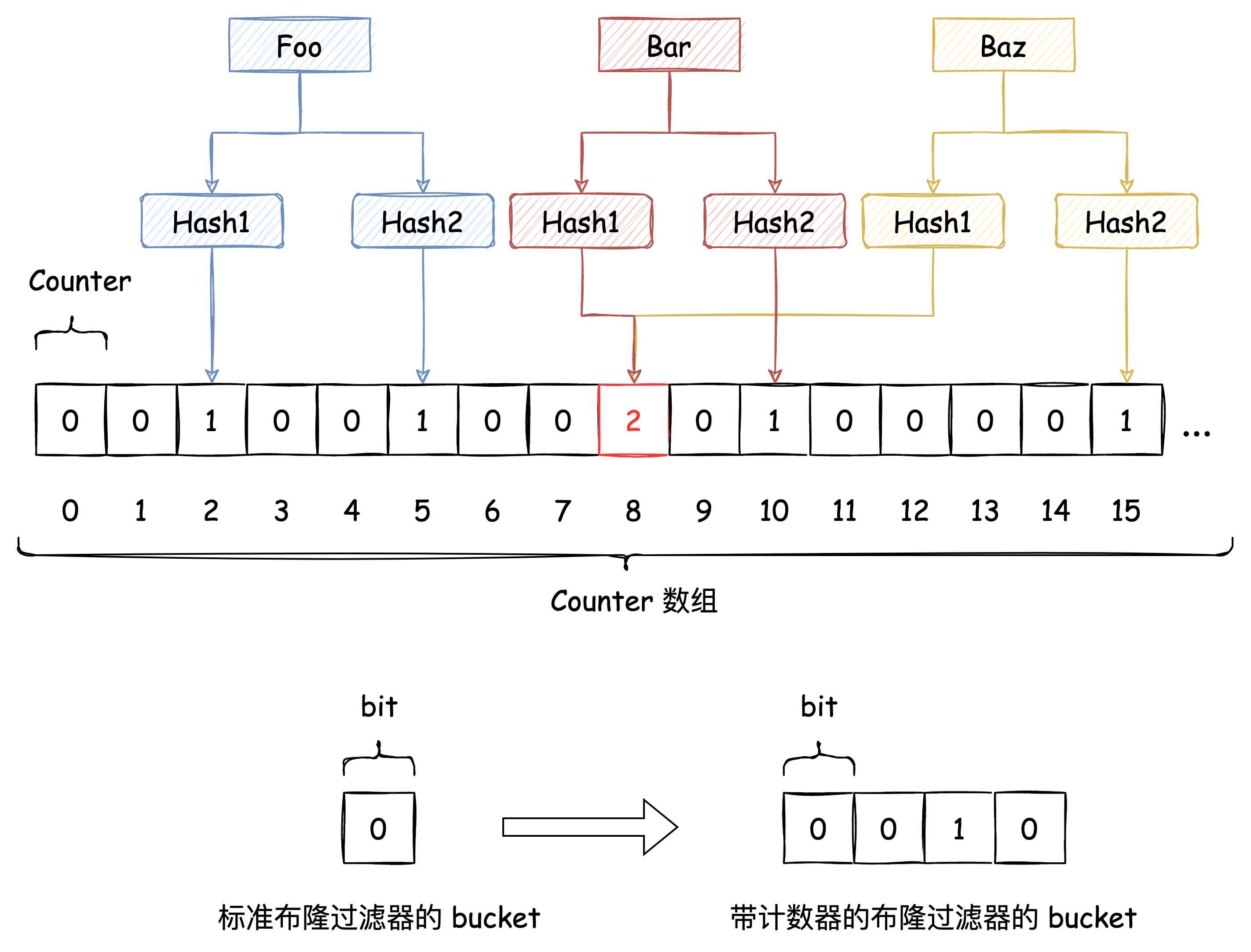## 分布式布隆过滤器实现方案

https://github.com/RedisBloom/RedisBloom

# 代码地址

posted @ 2022-06-26 22:29  黑洞视界  阅读(3716)  评论(16编辑  收藏  举报